# NCERT Exemplar Solutions for Class 12 Maths Chapter 12 Linear Programming

The NCERT Exemplar textbooks are important for students of the CBSE board in order to prepare for their exams. They contain various problems to strengthen the fundamental concepts of all the chapters. Students can further use these resources for revision before the board exam. The NCERT Exemplar Solutions are available subject-wise to clear any doubts regarding the concepts and problems in textbooks. Further, all the solutions are created following the latest CBSE guidelines.

The 12th Chapter of NCERT Exemplar Solutions for Class 12 Mathematics is Linear Programming. Topics included are linear programming problems and its mathematical formulation, the concept of optimising function, finding the maximum and minimum value of the given function, the graphical method of solving linear programming problems, and different types of linear programming problems – manufacturing problems, diet problems and transportation problems. To help students understand the methods of solving the exercise problems of this chapter, the solutions PDF of NCERT Exemplar Solutions for Class 12 Maths Chapter 12 Linear Programming is available in the link below.

## Download the PDF of NCERT Exemplar Solutions for Class 12 Maths Chapter 12 Linear Programming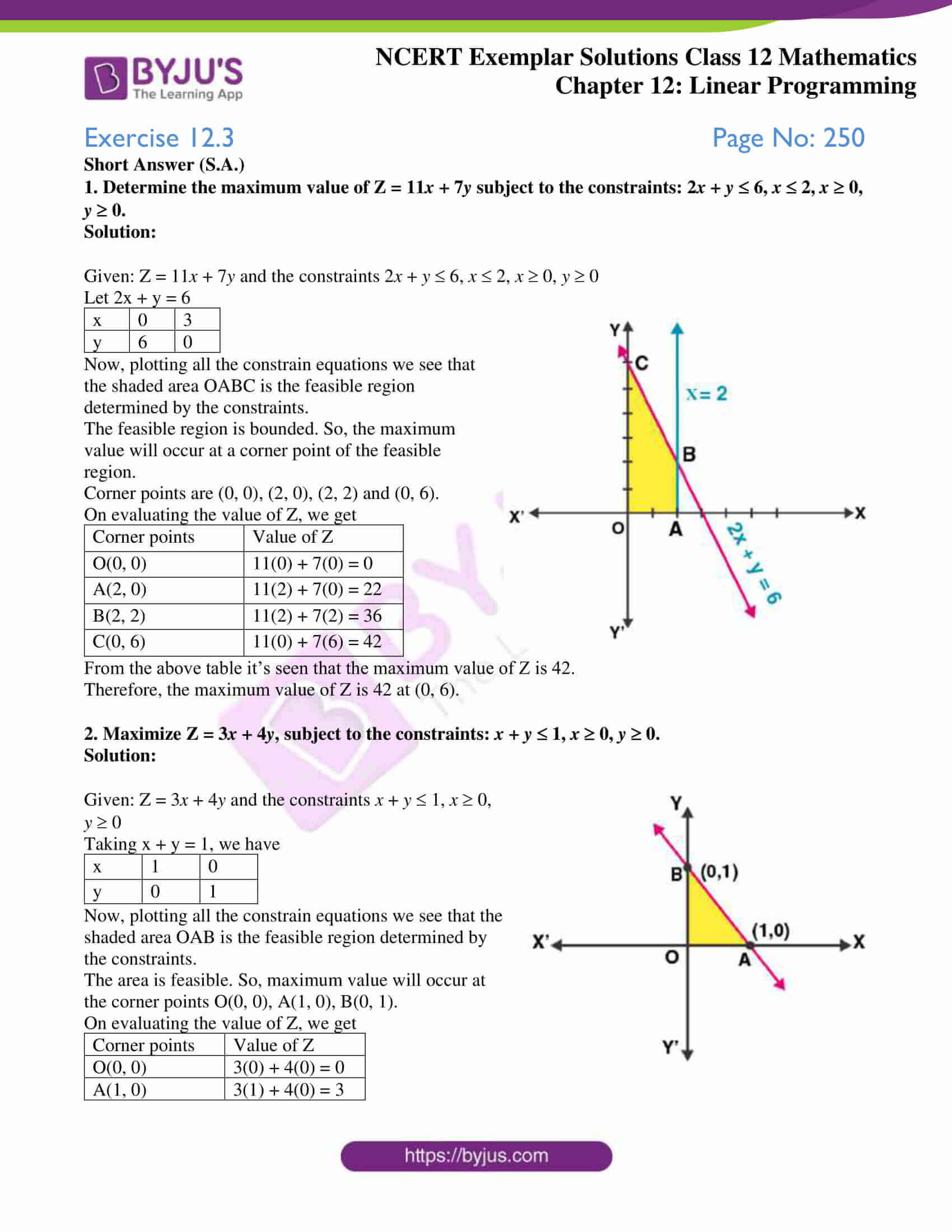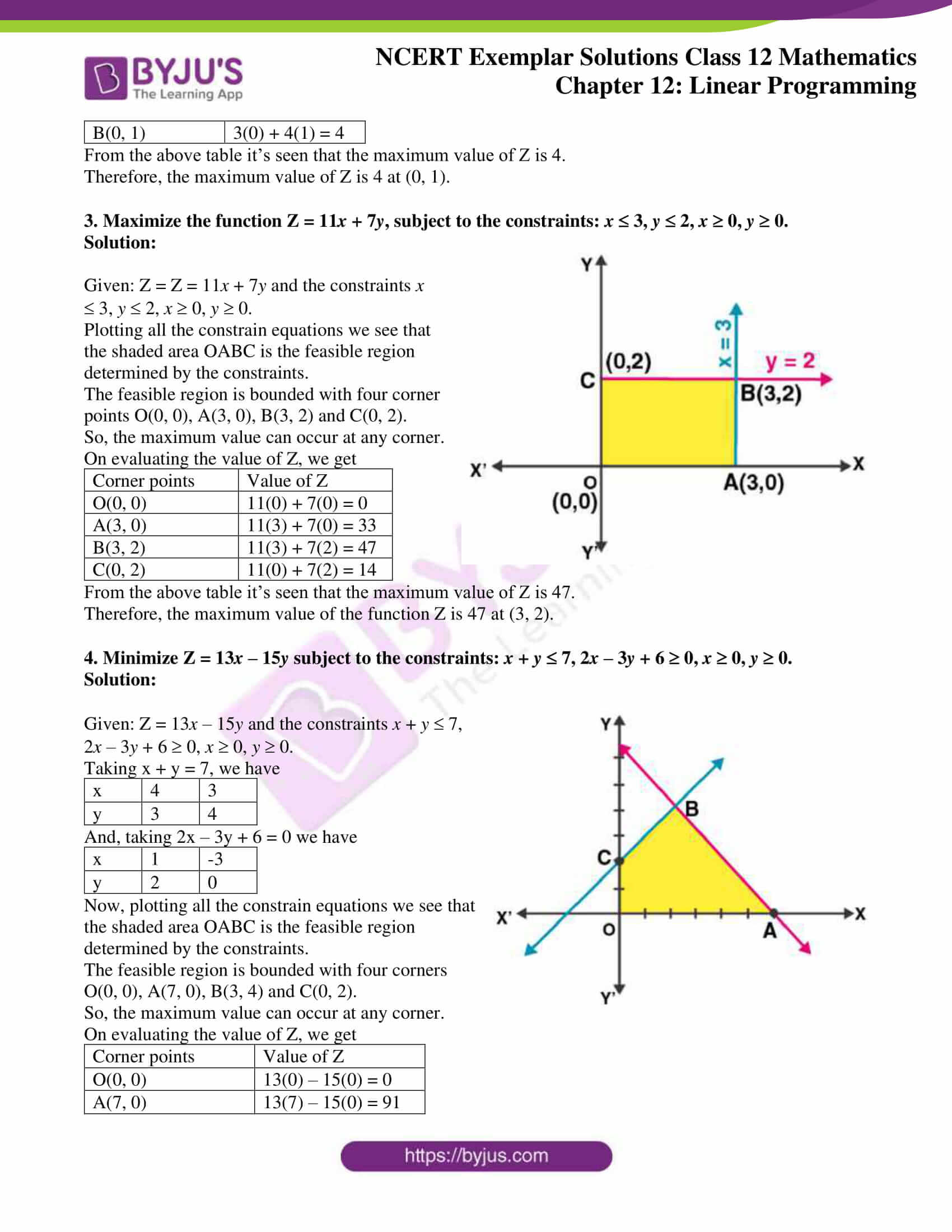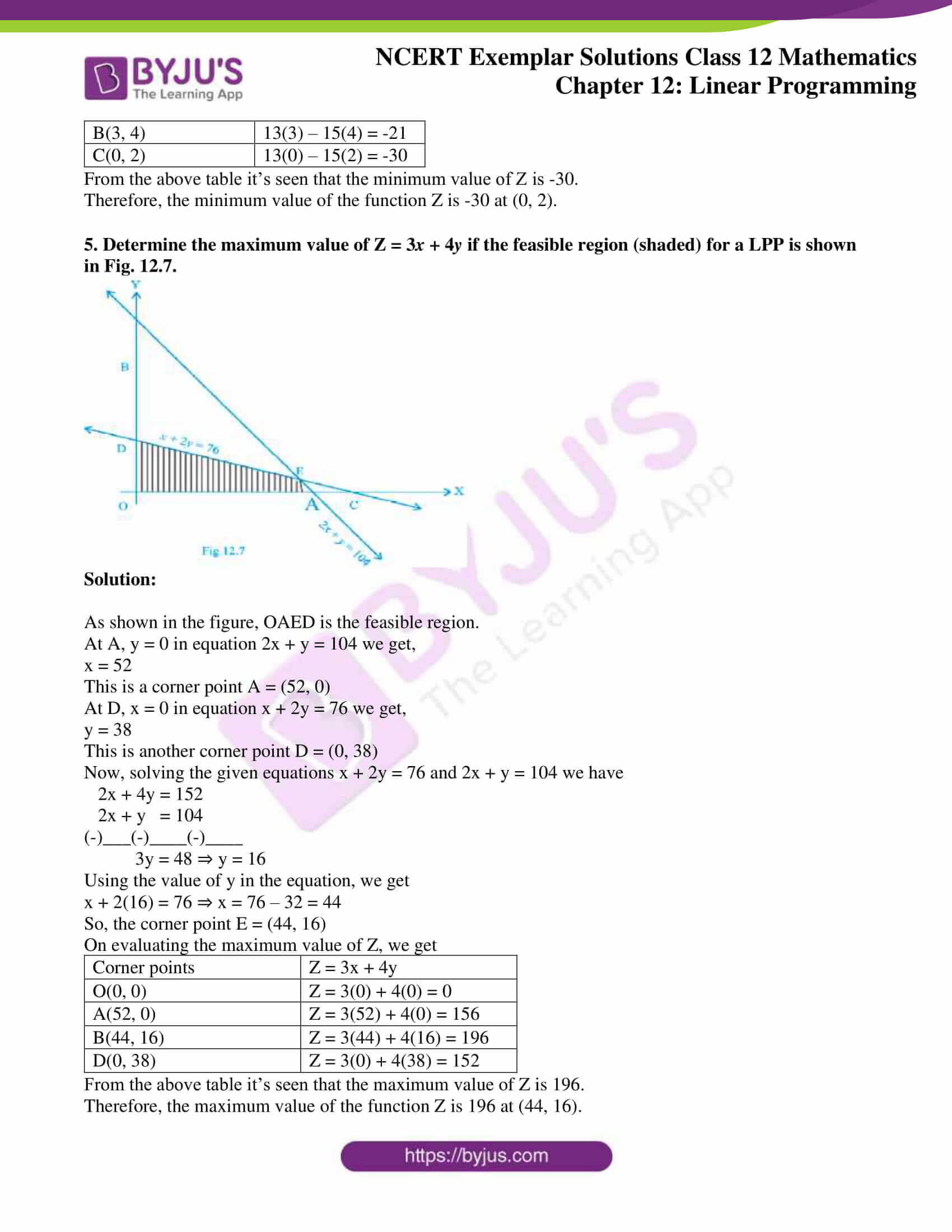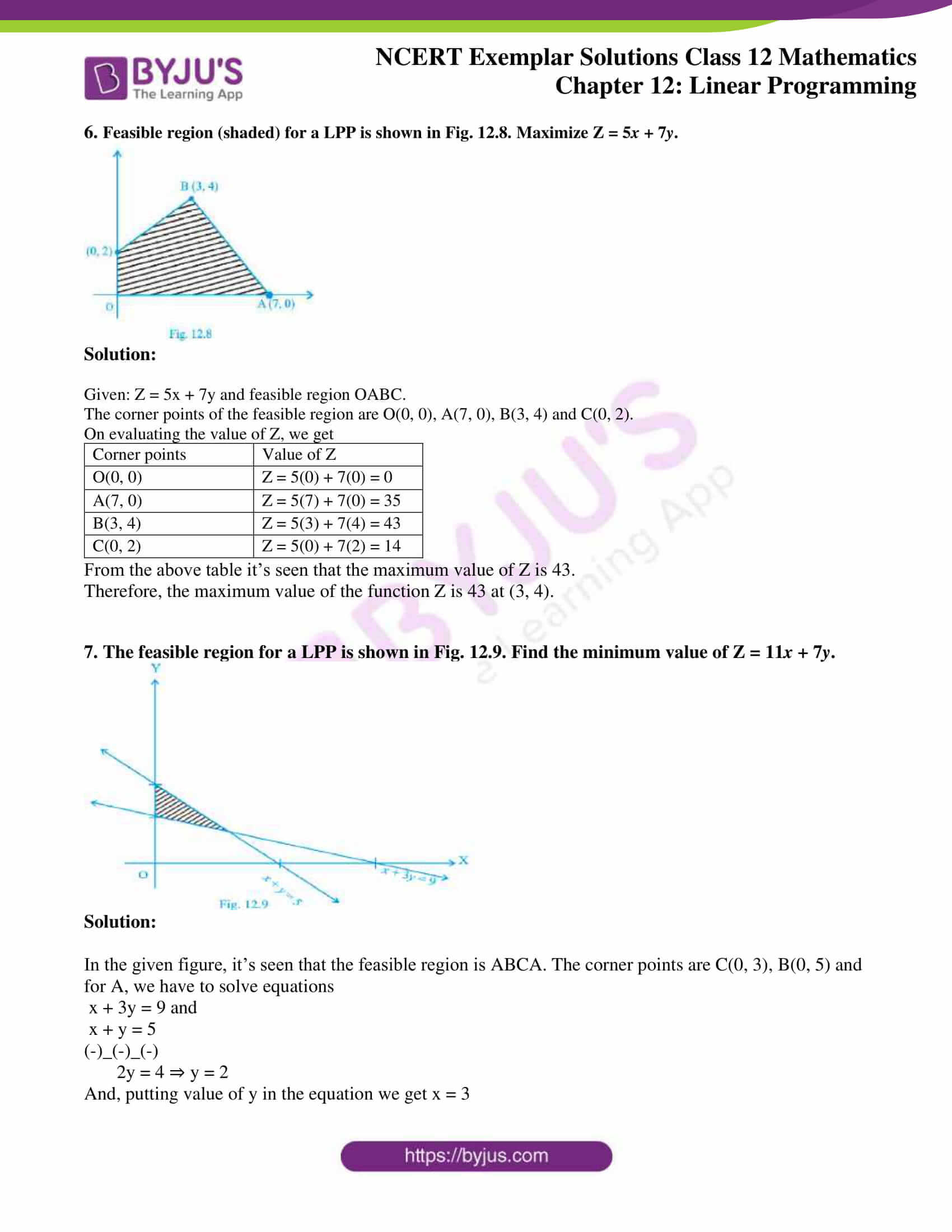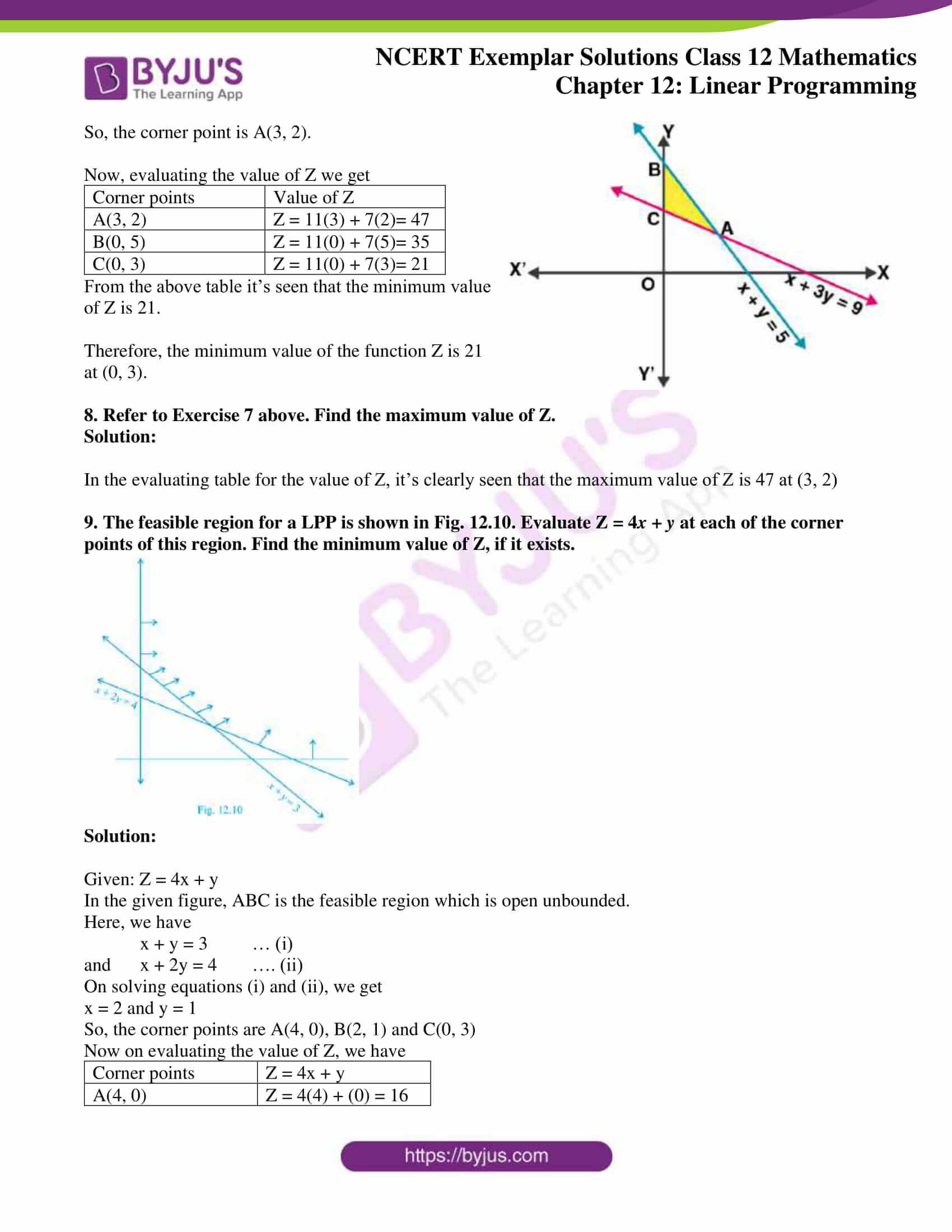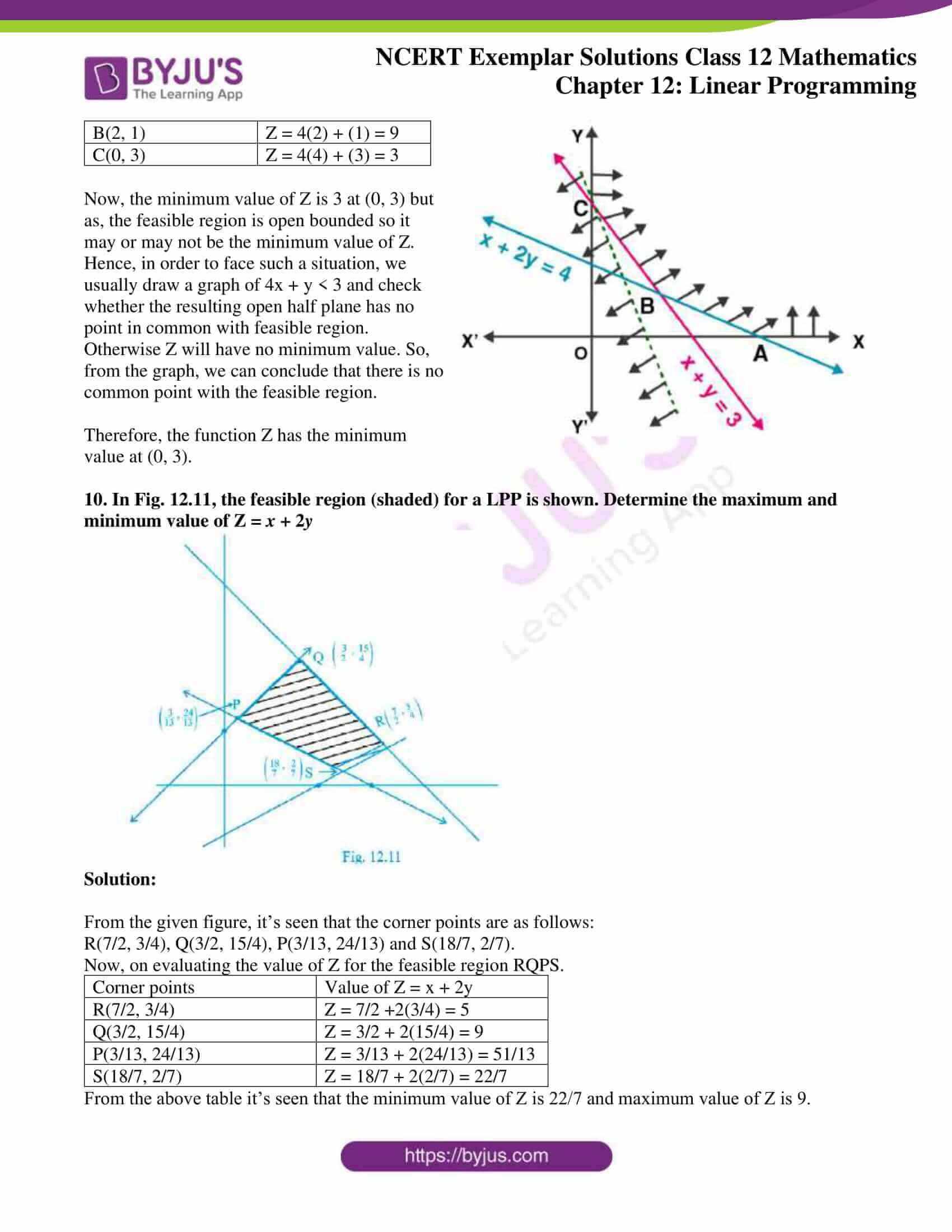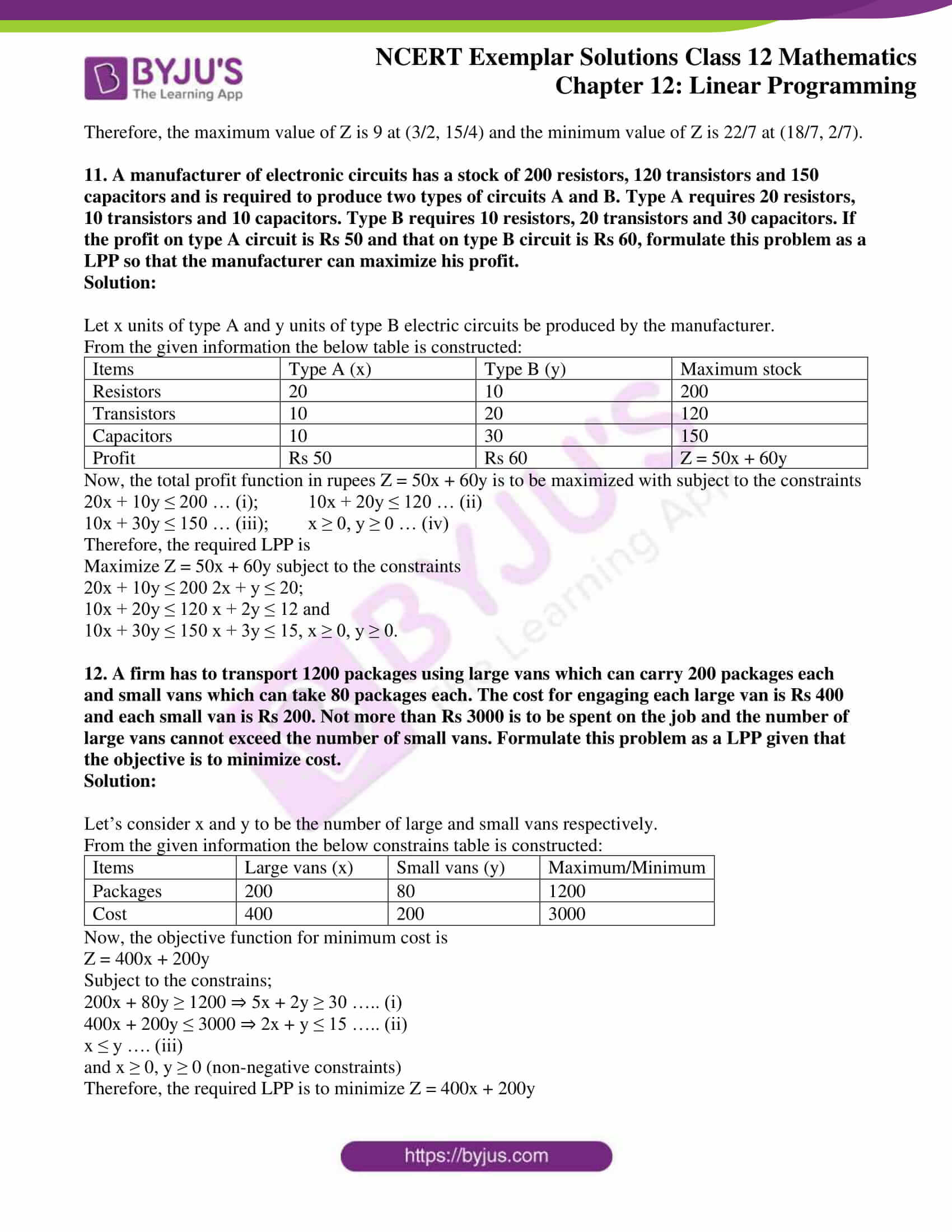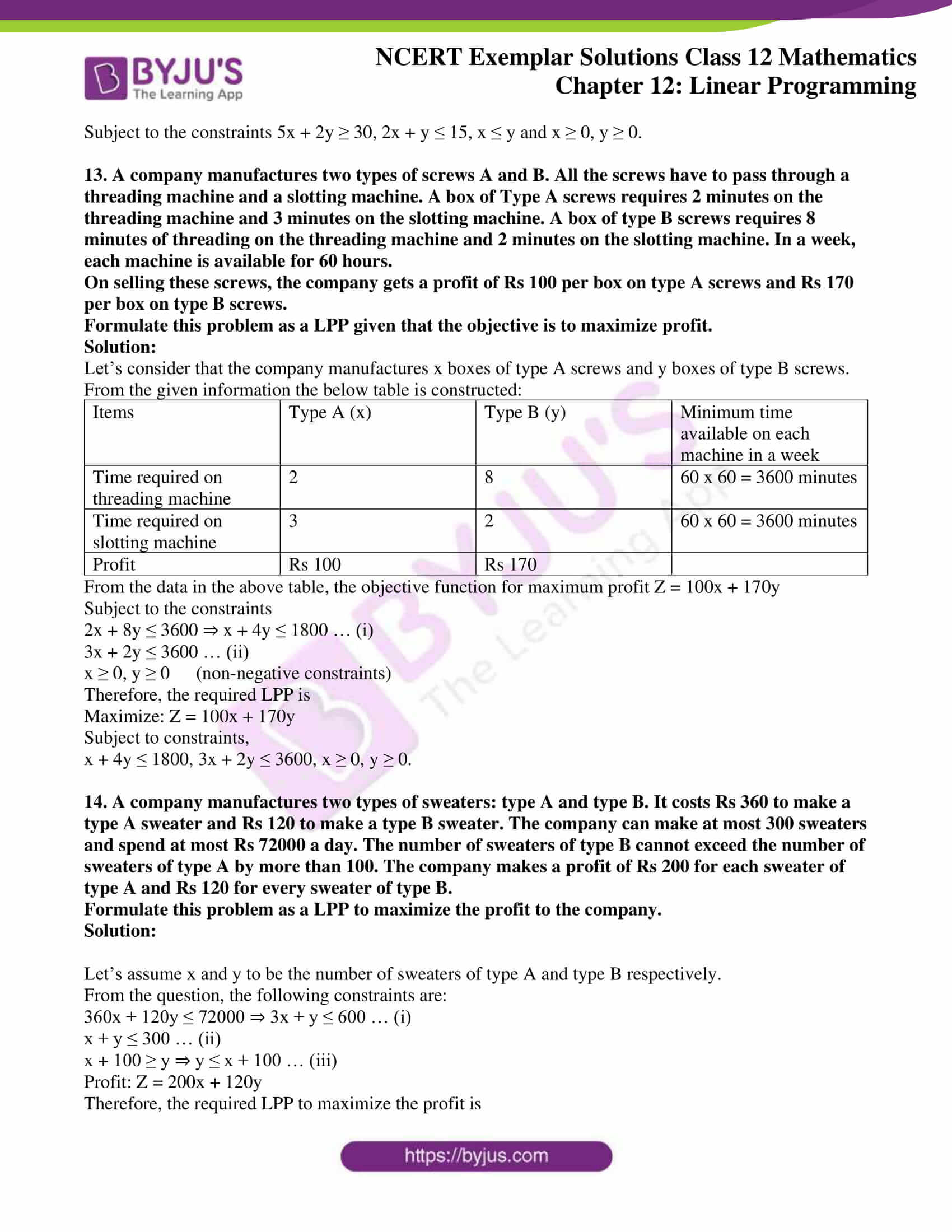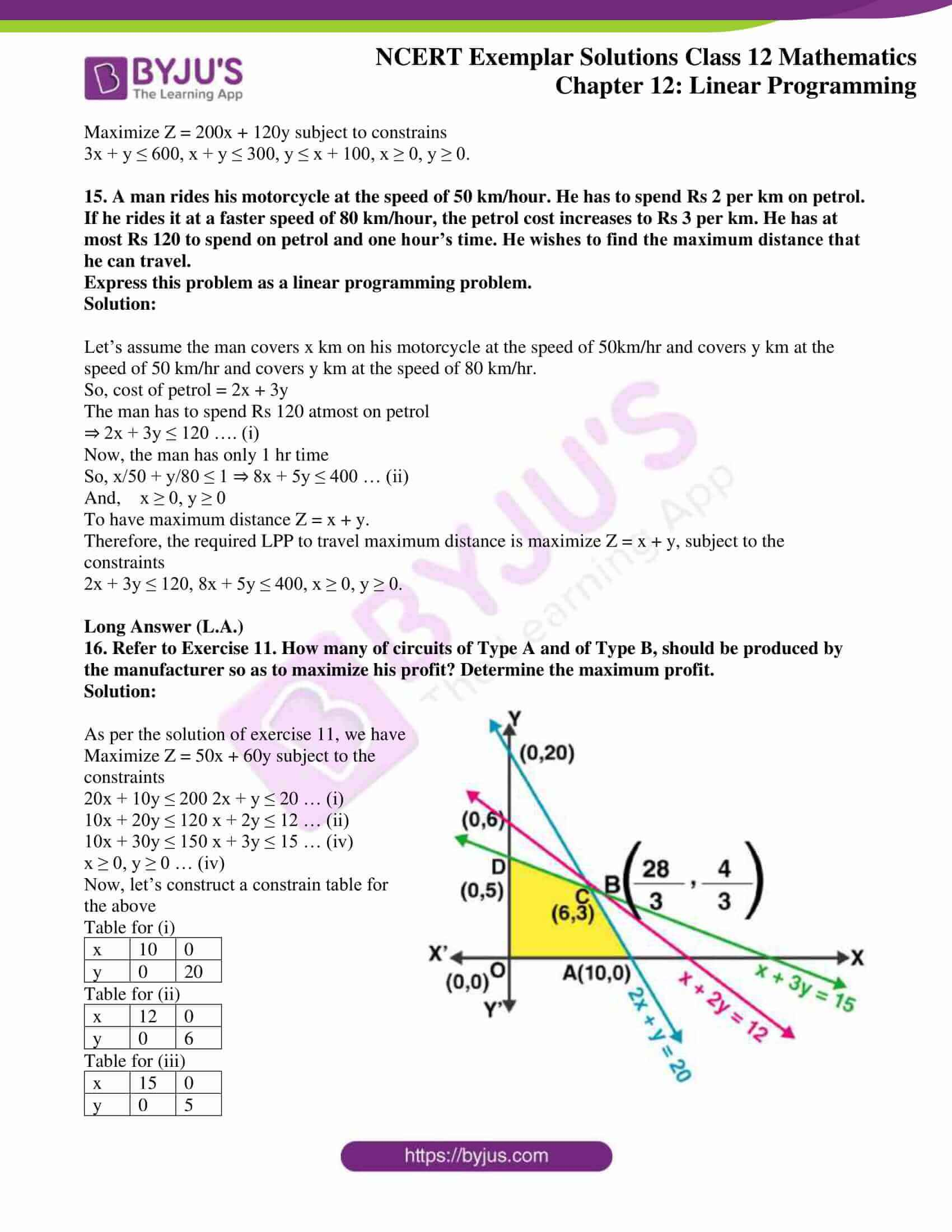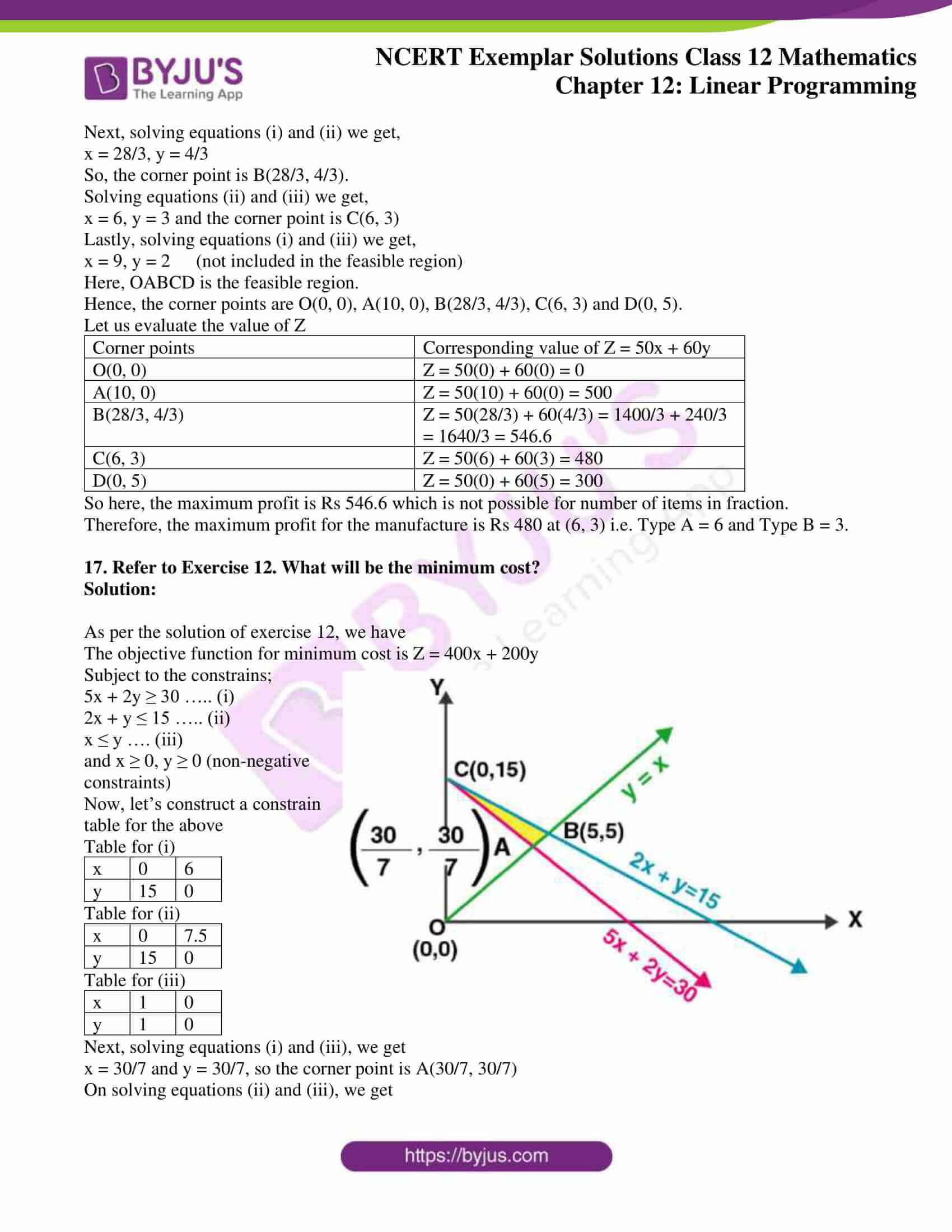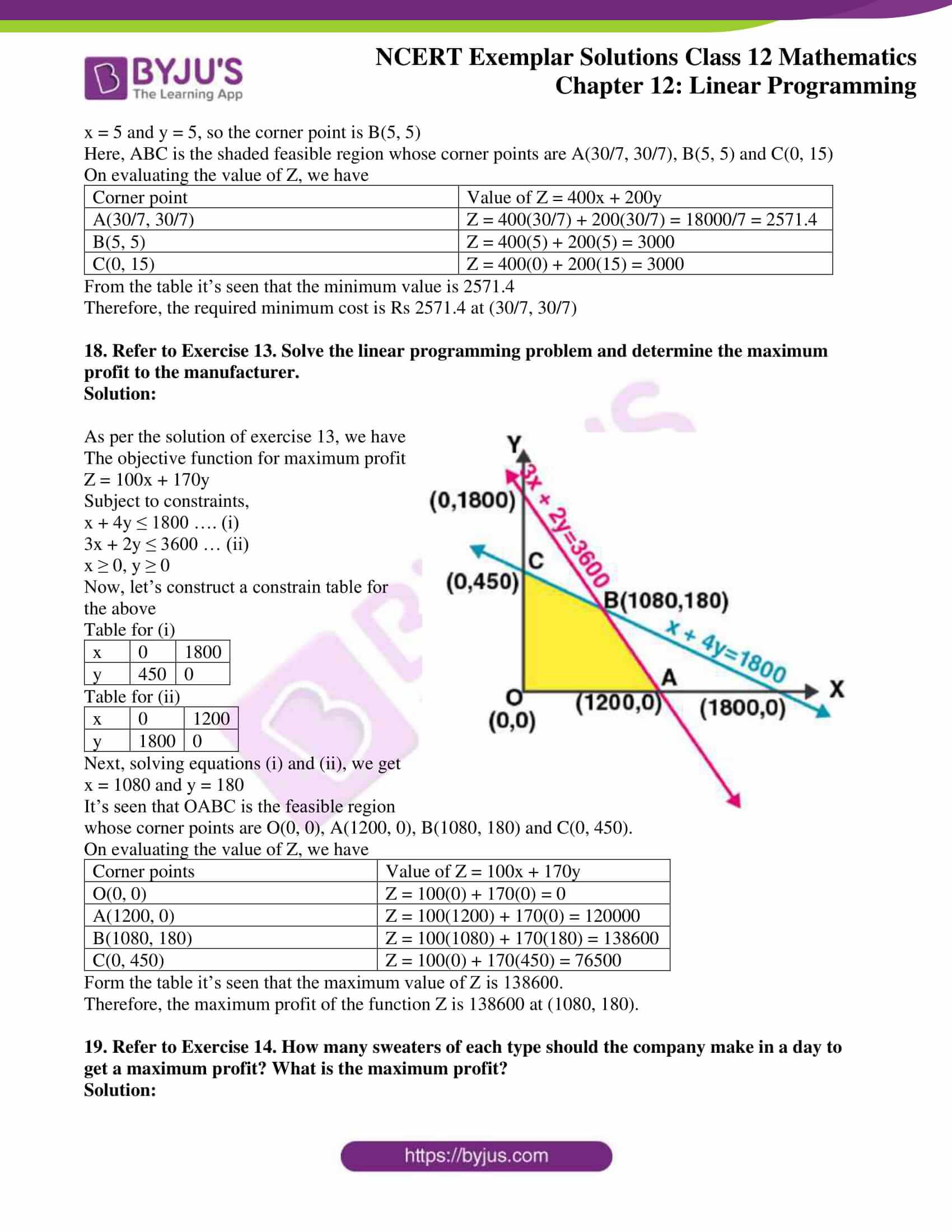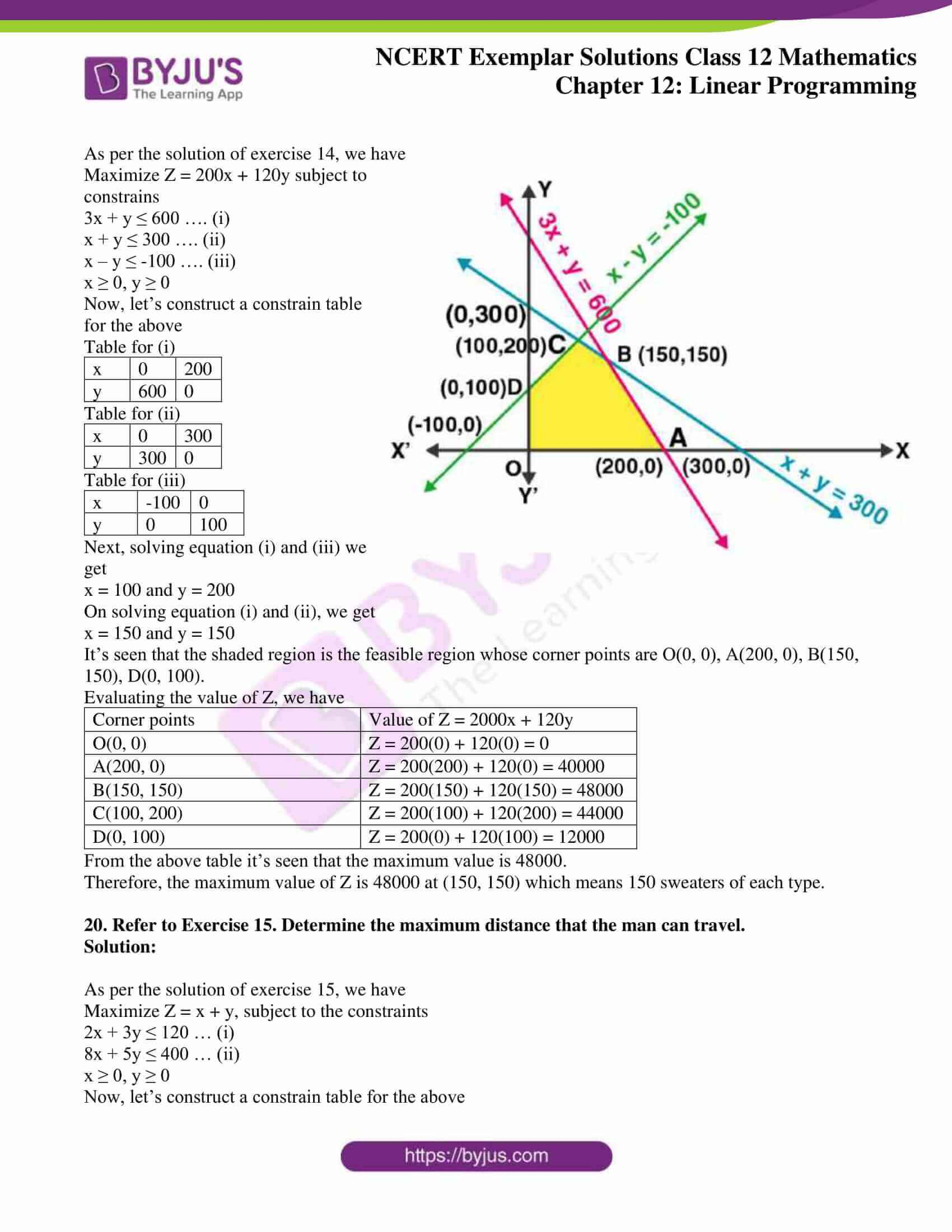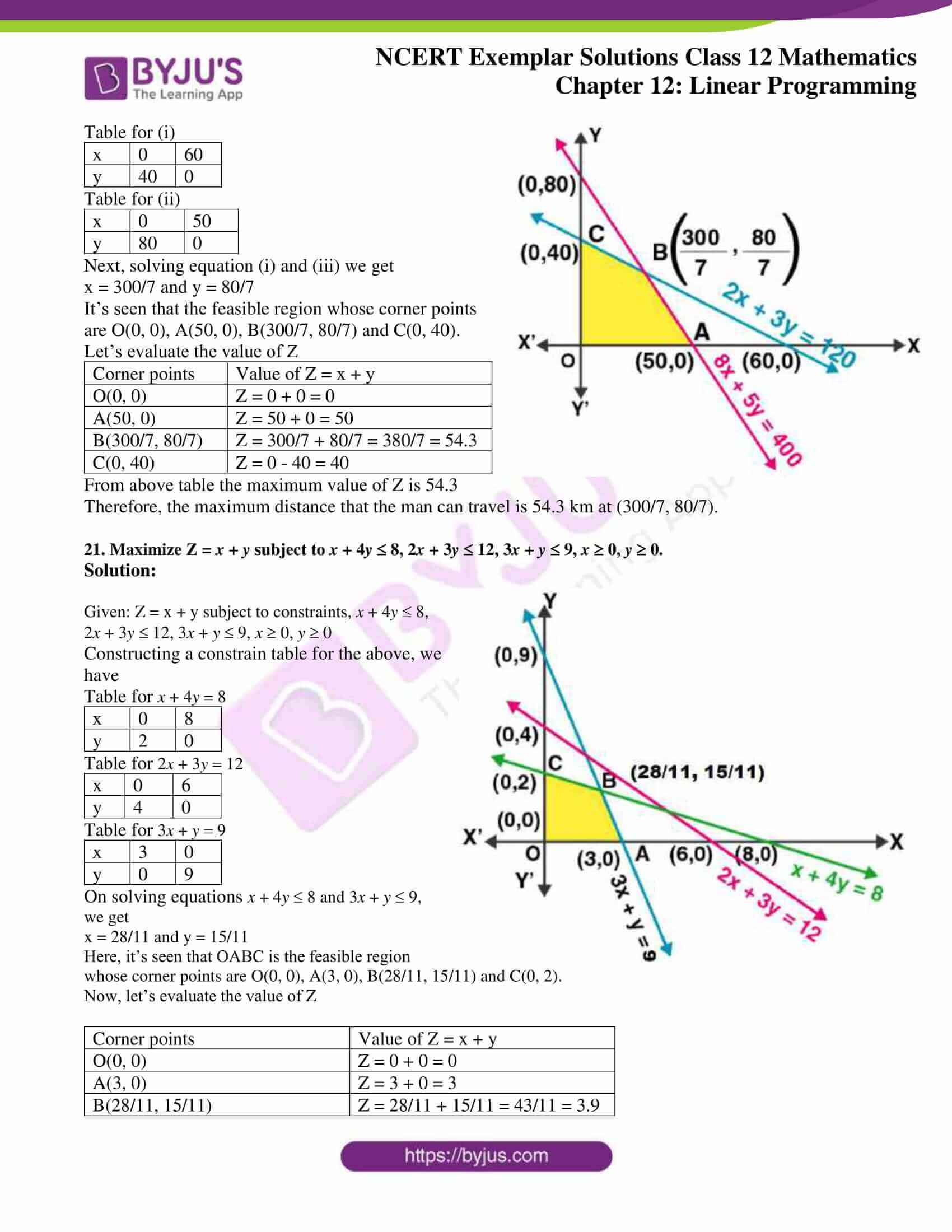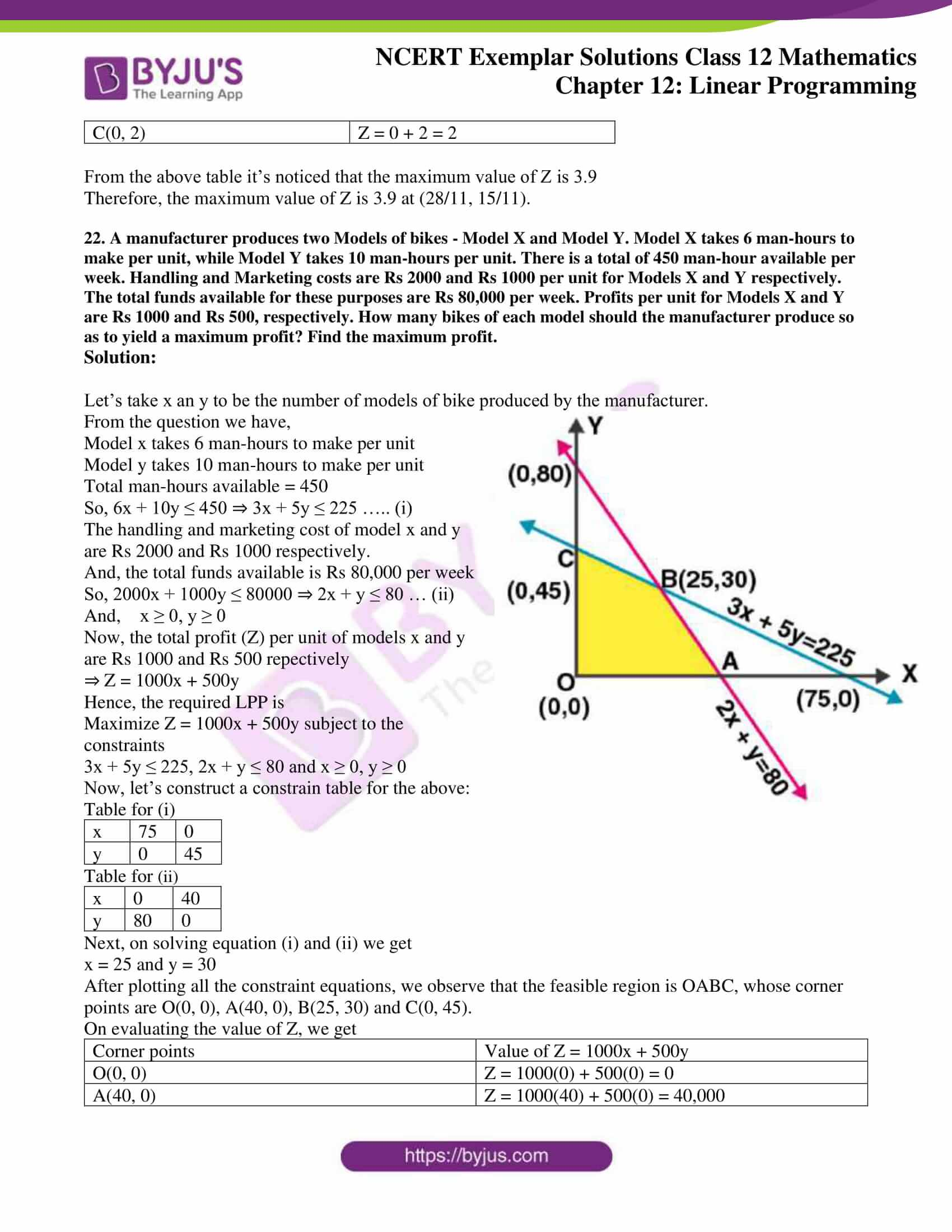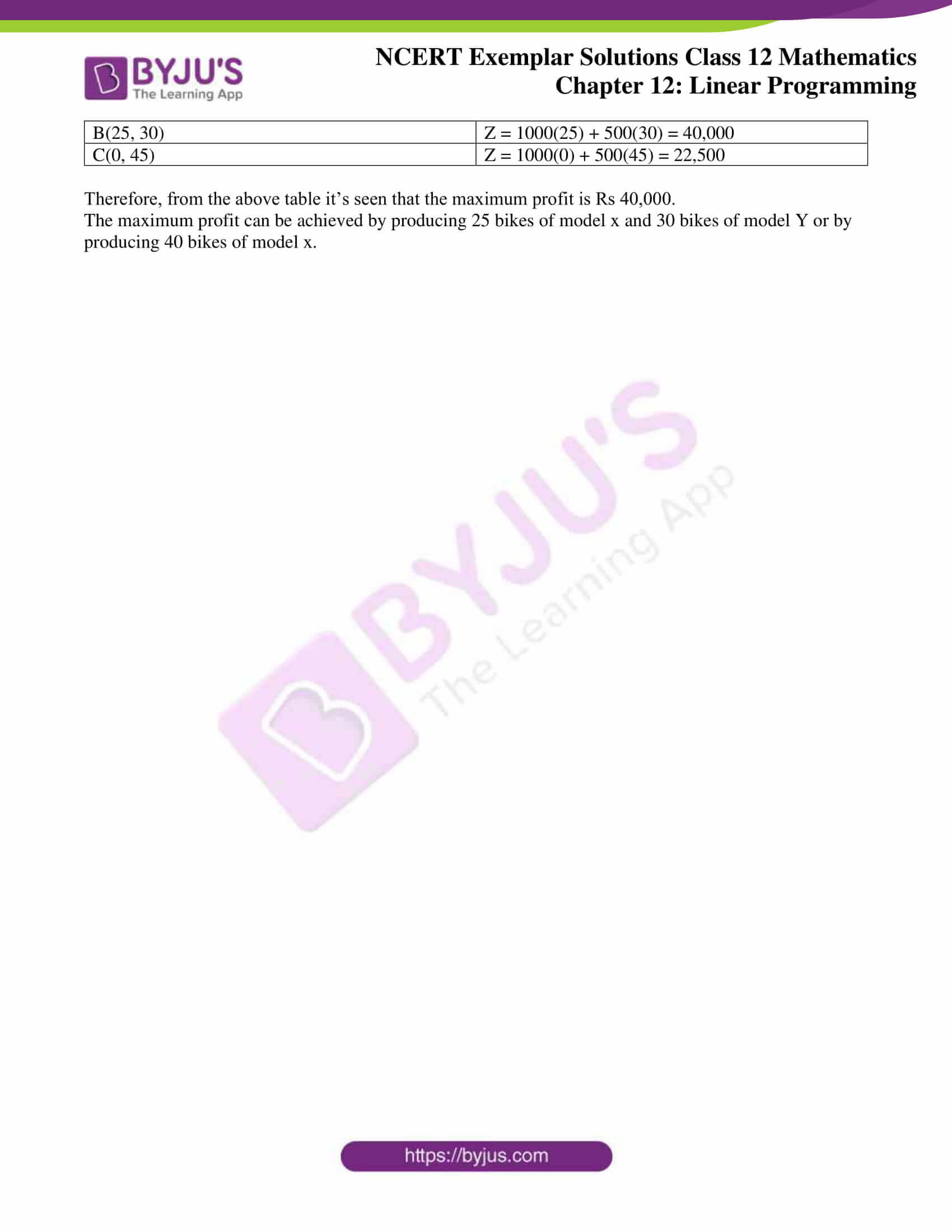### Access Answers to NCERT Exemplar Solutions for Class 12 Maths Chapter 12 Linear Programming

Exercise 12.3 Page No: 250

1. Determine the maximum value of Z = 11x + 7y subject to the constraints: 2x + y £ 6, x £ 2, x ³ 0, y ³ 0.

Solution:

Given: Z = 11x + 7y and the constraints 2x + y £ 6, x £ 2, x ³ 0, y ³ 0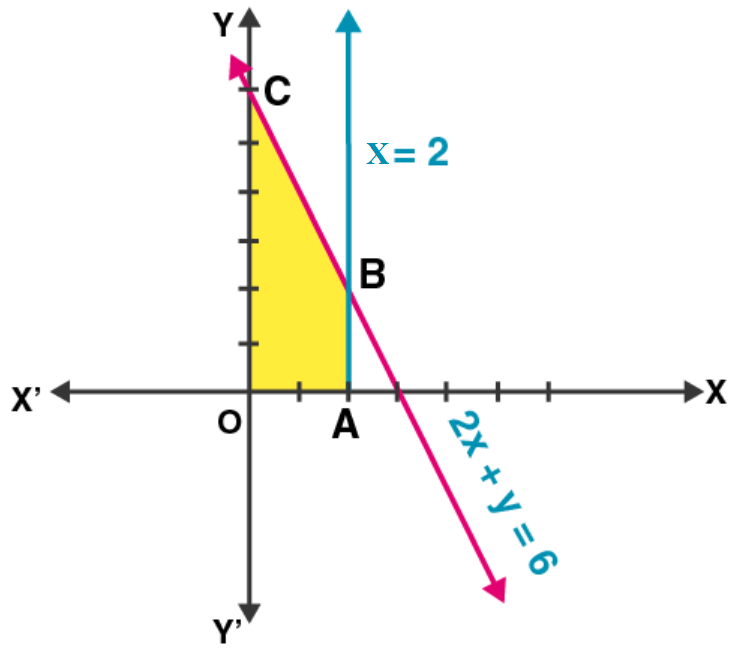Let 2x + y = 6

 x 0 3 y 6 0

Now, plotting all the constrain equations we see that the shaded area OABC is the feasible region determined by the constraints.

The feasible region is bounded. So, the maximum value will occur at a corner point of the feasible region.

Corner points are (0, 0), (2, 0), (2, 2) and (0, 6).

On evaluating the value of Z, we get

 Corner points Value of Z O(0, 0) 11(0) + 7(0) = 0 A(2, 0) 11(2) + 7(0) = 22 B(2, 2) 11(2) + 7(2) = 36 C(0, 6) 11(0) + 7(6) = 42

From the above table it’s seen that the maximum value of Z is 42.

Therefore, the maximum value of Z is 42 at (0, 6).

2. Maximize Z = 3x + 4y, subject to the constraints: x + y £ 1, x ³ 0, y ³ 0.

Solution: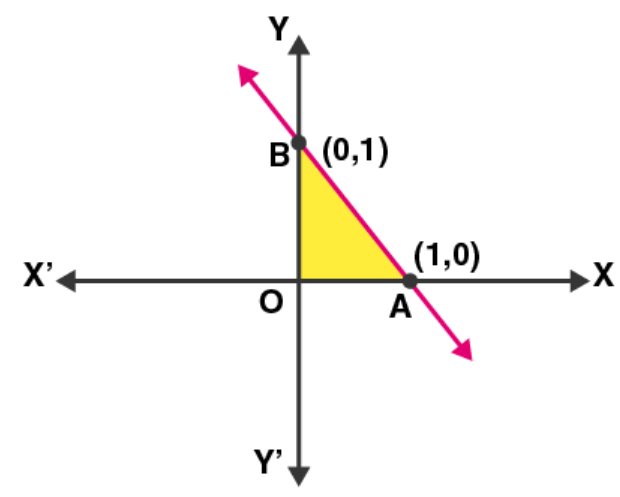Given: Z = 3x + 4y and the constraints x + y £ 1, x ³ 0,

y ³ 0

Taking x + y = 1, we have

 x 1 0 y 0 1

Now, plotting all the constrain equations we see that the shaded area OAB is the feasible region determined by the constraints.

The area is feasible. So, maximum value will occur at the corner points O(0, 0), A(1, 0), B(0, 1).

On evaluating the value of Z, we get

 Corner points Value of Z O(0, 0) 3(0) + 4(0) = 0 A(1, 0) 3(1) + 4(0) = 3 B(0, 1) 3(0) + 4(1) = 4

From the above table it’s seen that the maximum value of Z is 4.

Therefore, the maximum value of Z is 4 at (0, 1).

3. Maximize the function Z = 11x + 7y, subject to the constraints: x £ 3, y £ 2, x ³ 0, y ³ 0.

Solution: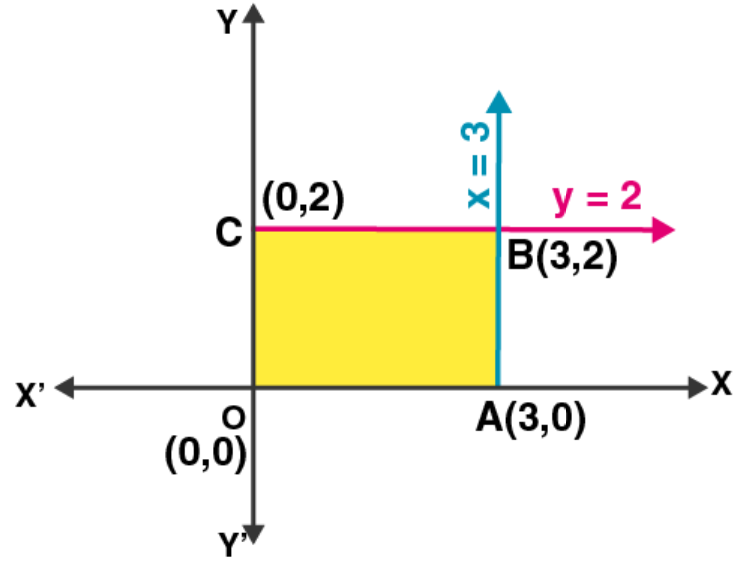Given: Z = Z = 11x + 7y and the constraints x £ 3, y £ 2, x ³ 0, y ³ 0.

Plotting all the constrain equations we see that the shaded area OABC is the feasible region determined by the constraints.

The feasible region is bounded with four corner points O(0, 0), A(3, 0), B(3, 2) and C(0, 2).

So, the maximum value can occur at any corner.

On evaluating the value of Z, we get

 Corner points Value of Z O(0, 0) 11(0) + 7(0) = 0 A(3, 0) 11(3) + 7(0) = 33 B(3, 2) 11(3) + 7(2) = 47 C(0, 2) 11(0) + 7(2) = 14

From the above table it’s seen that the maximum value of Z is 47.

Therefore, the maximum value of the function Z is 47 at (3, 2).

4. Minimize Z = 13x – 15y subject to the constraints: x + y £ 7, 2x – 3y + 6 ³ 0, x ³ 0, y ³ 0.

Solution: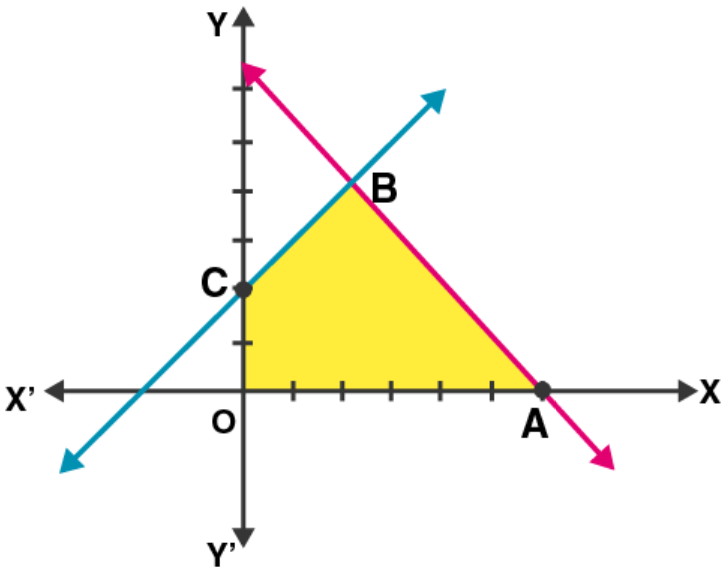Given: Z = 13x – 15y and the constraints x + y £ 7, 2x – 3y + 6 ³ 0, x ³ 0, y ³ 0.

Taking x + y = 7, we have

 x 4 3 y 3 4

And, taking 2x – 3y + 6 = 0 we have

 x 1 -3 y 2 0

Now, plotting all the constrain equations we see that the shaded area OABC is the feasible region determined by the constraints.

The feasible region is bounded with four corners O(0, 0), A(7, 0), B(3, 4) and C(0, 2).

So, the maximum value can occur at any corner.

On evaluating the value of Z, we get

 Corner points Value of Z O(0, 0) 13(0) – 15(0) = 0 A(7, 0) 13(7) – 15(0) = 91 B(3, 4) 13(3) – 15(4) = -21 C(0, 2) 13(0) – 15(2) = -30

From the above table it’s seen that the minimum value of Z is -30.

Therefore, the minimum value of the function Z is -30 at (0, 2).

5. Determine the maximum value of Z = 3x + 4y if the feasible region (shaded) for a LPP is shown in Fig. 12.7.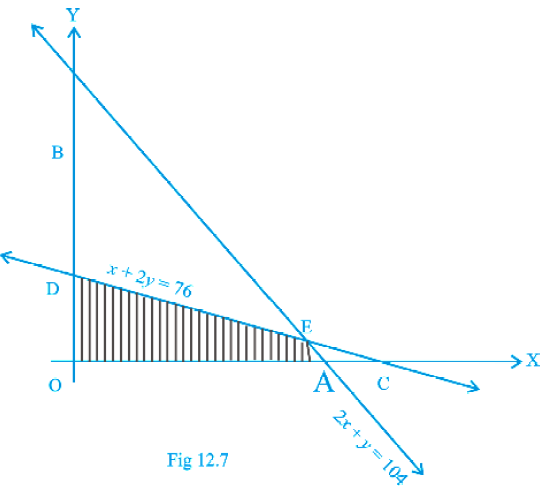Solution:

As shown in the figure, OAED is the feasible region.

At A, y = 0 in equation 2x + y = 104 we get,

x = 52

This is a corner point A = (52, 0)

At D, x = 0 in equation x + 2y = 76 we get,

y = 38

This is another corner point D = (0, 38)

Now, solving the given equations x + 2y = 76 and 2x + y = 104 we have

2x + 4y = 152

2x + y = 104

(-)___(-)____(-)____

3y = 48 ⇒ y = 16

Using the value of y in the equation, we get

x + 2(16) = 76 ⇒ x = 76 – 32 = 44

So, the corner point E = (44, 16)

On evaluating the maximum value of Z, we get

 Corner points Z = 3x + 4y O(0, 0) Z = 3(0) + 4(0) = 0 A(52, 0) Z = 3(52) + 4(0) = 156 B(44, 16) Z = 3(44) + 4(16) = 196 D(0, 38) Z = 3(0) + 4(38) = 152

From the above table it’s seen that the maximum value of Z is 196.

Therefore, the maximum value of the function Z is 196 at (44, 16).

6. Feasible region (shaded) for a LPP is shown in Fig. 12.8. Maximize Z = 5x + 7y.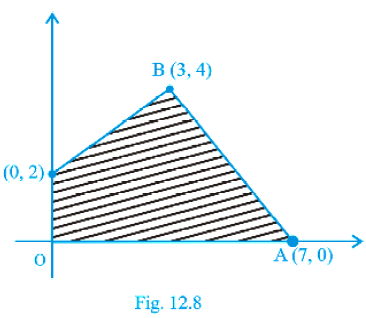Solution:

Given: Z = 5x + 7y and feasible region OABC.

The corner points of the feasible region are O(0, 0), A(7, 0), B(3, 4) and C(0, 2).

On evaluating the value of Z, we get

 Corner points Value of Z O(0, 0) Z = 5(0) + 7(0) = 0 A(7, 0) Z = 5(7) + 7(0) = 35 B(3, 4) Z = 5(3) + 7(4) = 43 C(0, 2) Z = 5(0) + 7(2) = 14

From the above table it’s seen that the maximum value of Z is 43.

Therefore, the maximum value of the function Z is 43 at (3, 4).

7. The feasible region for a LPP is shown in Fig. 12.9. Find the minimum value of Z = 11x + 7y.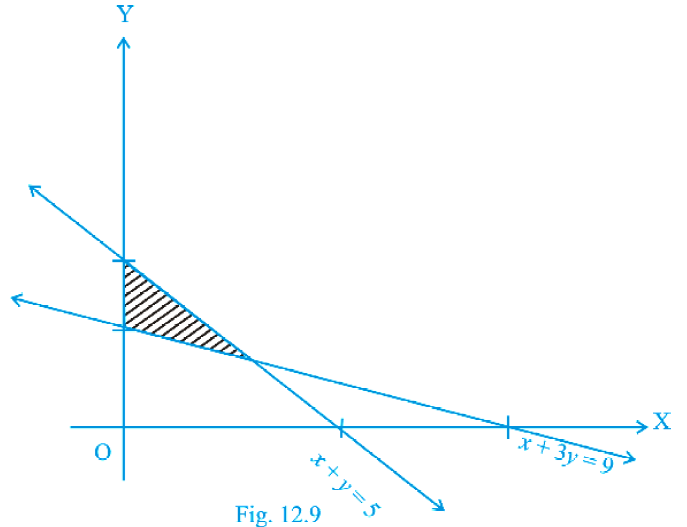Solution:

In the given figure, it’s seen that the feasible region is ABCA. The corner points are C(0, 3), B(0, 5) and for A, we have to solve equations

x + 3y = 9 and

x + y = 5

(-)_(-)_(-)

2y = 4 ⇒ y = 2

And, putting value of y in the equation we get x = 3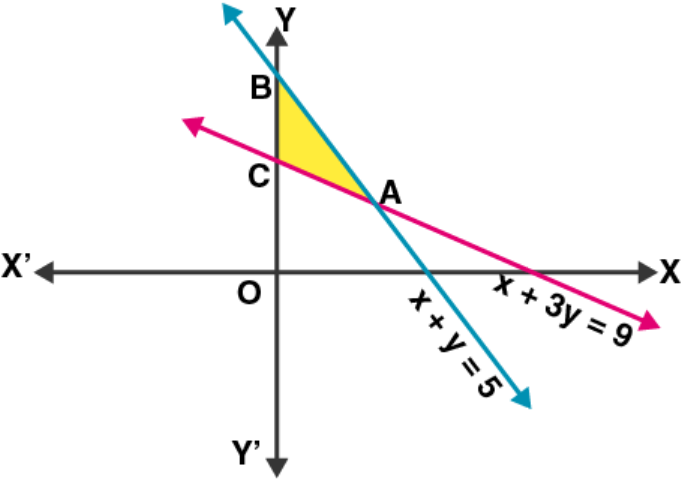So, the corner point is A(3, 2).

Now, evaluating the value of Z we get

 Corner points Value of Z A(3, 2) Z = 11(3) + 7(2)= 47 B(0, 5) Z = 11(0) + 7(5)= 35 C(0, 3) Z = 11(0) + 7(3)= 21

From the above table it’s seen that the minimum value of Z is 21.

Therefore, the minimum value of the function Z is 21 at (0, 3).

8. Refer to Exercise 7 above. Find the maximum value of Z.

Solution:

In the evaluating table for the value of Z, it’s clearly seen that the maximum value of Z is 47 at (3, 2)

9. The feasible region for a LPP is shown in Fig. 12.10. Evaluate Z = 4x + y at each of the corner points of this region. Find the minimum value of Z, if it exists.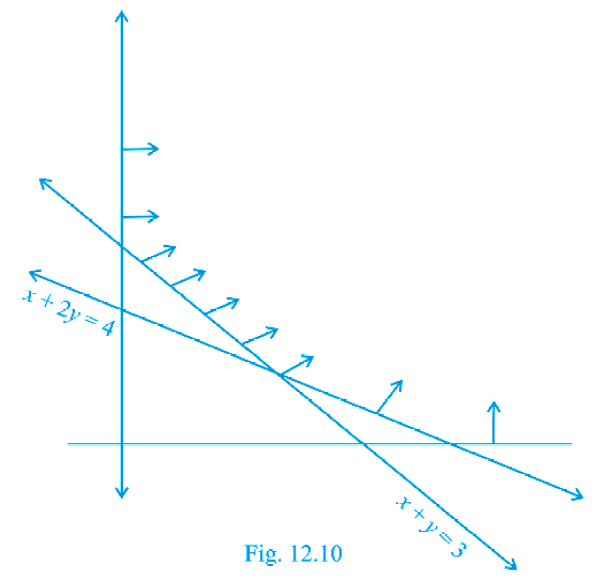Solution:

Given: Z = 4x + y

In the given figure, ABC is the feasible region which is open unbounded.

Here, we have

x + y = 3 … (i)

and x + 2y = 4 …. (ii)

On solving equations (i) and (ii), we get

x = 2 and y = 1

So, the corner points are A(4, 0), B(2, 1) and C(0, 3)

Now on evaluating the value of Z, we have

 Corner points Z = 4x + y A(4, 0) Z = 4(4) + (0) = 16 B(2, 1) Z = 4(2) + (1) = 9 C(0, 3) Z = 4(4) + (3) = 3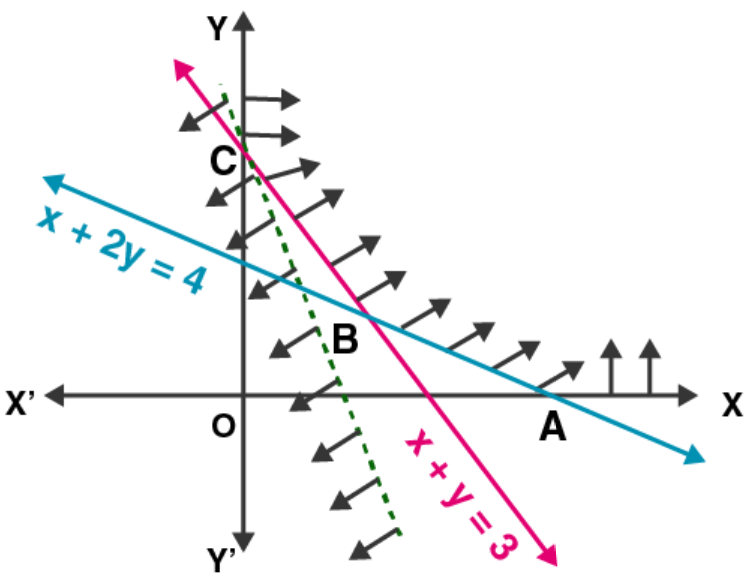Now, the minimum value of Z is 3 at (0, 3) but as, the feasible region is open bounded so it may or may not be the minimum value of Z.

Hence, in order to face such a situation, we usually draw a graph of 4x + y < 3 and check whether the resulting open half plane has no point in common with feasible region. Otherwise Z will have no minimum value. So, from the graph, we can conclude that there is no common point with the feasible region.

Therefore, the function Z has the minimum value at (0, 3).

10. In Fig. 12.11, the feasible region (shaded) for a LPP is shown. Determine the maximum and minimum value of Z = x + 2y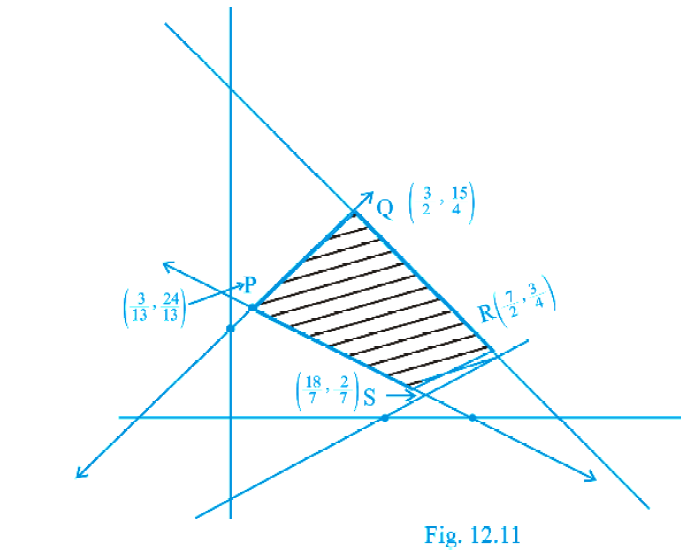Solution:

From the given figure, it’s seen that the corner points are as follows:

R(7/2, 3/4), Q(3/2, 15/4), P(3/13, 24/13) and S(18/7, 2/7).

Now, on evaluating the value of Z for the feasible region RQPS.

 Corner points Value of Z = x + 2y R(7/2, 3/4) Z = 7/2 +2(3/4) = 5 Q(3/2, 15/4) Z = 3/2 + 2(15/4) = 9 P(3/13, 24/13) Z = 3/13 + 2(24/13) = 51/13 S(18/7, 2/7) Z = 18/7 + 2(2/7) = 22/7

From the above table it’s seen that the minimum value of Z is 22/7 and maximum value of Z is 9.

Therefore, the maximum value of Z is 9 at (3/2, 15/4) and the minimum value of Z is 22/7 at (18/7, 2/7).

11. A manufacturer of electronic circuits has a stock of 200 resistors, 120 transistors and 150 capacitors and is required to produce two types of circuits A and B. Type A requires 20 resistors, 10 transistors and 10 capacitors. Type B requires 10 resistors, 20 transistors and 30 capacitors. If the profit on type A circuit is Rs 50 and that on type B circuit is Rs 60, formulate this problem as a LPP so that the manufacturer can maximize his profit.

Solution:

Let x units of type A and y units of type B electric circuits be produced by the manufacturer.

From the given information the below table is constructed:

 Items Type A (x) Type B (y) Maximum stock Resistors 20 10 200 Transistors 10 20 120 Capacitors 10 30 150 Profit Rs 50 Rs 60 Z = 50x + 60y

Now, the total profit function in rupees Z = 50x + 60y is to be maximized with subject to the constraints

20x + 10y ≤ 200 … (i); 10x + 20y ≤ 120 … (ii)

10x + 30y ≤ 150 … (iii); x ≥ 0, y ≥ 0 … (iv)

Therefore, the required LPP is

Maximize Z = 50x + 60y subject to the constraints

20x + 10y ≤ 200 2x + y ≤ 20;

10x + 20y ≤ 120 x + 2y ≤ 12 and

10x + 30y ≤ 150 x + 3y ≤ 15, x ≥ 0, y ≥ 0.

12. A firm has to transport 1200 packages using large vans which can carry 200 packages each and small vans which can take 80 packages each. The cost for engaging each large van is Rs 400 and each small van is Rs 200. Not more than Rs 3000 is to be spent on the job and the number of large vans cannot exceed the number of small vans. Formulate this problem as a LPP given that

the objective is to minimize cost.

Solution:

Let’s consider x and y to be the number of large and small vans respectively.

From the given information the below constrains table is constructed:

 Items Large vans (x) Small vans (y) Maximum/Minimum Packages 200 80 1200 Cost 400 200 3000

Now, the objective function for minimum cost is

Z = 400x + 200y

Subject to the constrains;

200x + 80y ≥ 1200 ⇒ 5x + 2y ≥ 30 ….. (i)

400x + 200y ≤ 3000 ⇒ 2x + y ≤ 15 ….. (ii)

x ≤ y …. (iii)

and x ≥ 0, y ≥ 0 (non-negative constraints)

Therefore, the required LPP is to minimize Z = 400x + 200y

Subject to the constraints 5x + 2y ≥ 30, 2x + y ≤ 15, x ≤ y and x ≥ 0, y ≥ 0.

13. A company manufactures two types of screws A and B. All the screws have to pass through a threading machine and a slotting machine. A box of Type A screws requires 2 minutes on the threading machine and 3 minutes on the slotting machine. A box of type B screws requires 8 minutes of threading on the threading machine and 2 minutes on the slotting machine. In a week, each machine is available for 60 hours.

On selling these screws, the company gets a profit of Rs 100 per box on type A screws and Rs 170 per box on type B screws.

Formulate this problem as a LPP given that the objective is to maximize profit.

Solution:

Let’s consider that the company manufactures x boxes of type A screws and y boxes of type B screws.

From the given information the below table is constructed:

 Items Type A (x) Type B (y) Minimum time available on each machine in a week Time required on threading machine 2 8 60 x 60 = 3600 minutes Time required on slotting machine 3 2 60 x 60 = 3600 minutes Profit Rs 100 Rs 170

From the data in the above table, the objective function for maximum profit Z = 100x + 170y

Subject to the constraints

2x + 8y ≤ 3600 ⇒ x + 4y ≤ 1800 … (i)

3x + 2y ≤ 3600 … (ii)

x ≥ 0, y ≥ 0 (non-negative constraints)

Therefore, the required LPP is

Maximize: Z = 100x + 170y

Subject to constraints,

x + 4y ≤ 1800, 3x + 2y ≤ 3600, x ≥ 0, y ≥ 0.

14. A company manufactures two types of sweaters: type A and type B. It costs Rs 360 to make a type A sweater and Rs 120 to make a type B sweater. The company can make at most 300 sweaters and spend at most Rs 72000 a day. The number of sweaters of type B cannot exceed the number of sweaters of type A by more than 100. The company makes a profit of Rs 200 for each sweater of type A and Rs 120 for every sweater of type B.

Formulate this problem as a LPP to maximize the profit to the company.

Solution:

Let’s assume x and y to be the number of sweaters of type A and type B respectively.

From the question, the following constraints are:

360x + 120y ≤ 72000 ⇒ 3x + y ≤ 600 … (i)

x + y ≤ 300 … (ii)

x + 100 ≥ y ⇒ y ≤ x + 100 … (iii)

Profit: Z = 200x + 120y

Therefore, the required LPP to maximize the profit is

Maximize Z = 200x + 120y subject to constrains

3x + y ≤ 600, x + y ≤ 300, y ≤ x + 100, x ≥ 0, y ≥ 0.

15. A man rides his motorcycle at the speed of 50 km/hour. He has to spend Rs 2 per km on petrol. If he rides it at a faster speed of 80 km/hour, the petrol cost increases to Rs 3 per km. He has at most Rs 120 to spend on petrol and one hour’s time. He wishes to find the maximum distance that he can travel.

Express this problem as a linear programming problem.

Solution:

Let’s assume the man covers x km on his motorcycle at the speed of 50km/hr and covers y km at the speed of 50 km/hr and covers y km at the speed of 80 km/hr.

So, cost of petrol = 2x + 3y

The man has to spend Rs 120 atmost on petrol

⇒ 2x + 3y ≤ 120 …. (i)

Now, the man has only 1 hr time

So, x/50 + y/80 ≤ 1 ⇒ 8x + 5y ≤ 400 … (ii)

And, x ≥ 0, y ≥ 0

To have maximum distance Z = x + y.

Therefore, the required LPP to travel maximum distance is maximize Z = x + y, subject to the constraints

2x + 3y ≤ 120, 8x + 5y ≤ 400, x ≥ 0, y ≥ 0.

16. Refer to Exercise 11. How many of circuits of Type A and of Type B, should be produced by the manufacturer so as to maximize his profit? Determine the maximum profit.

Solution: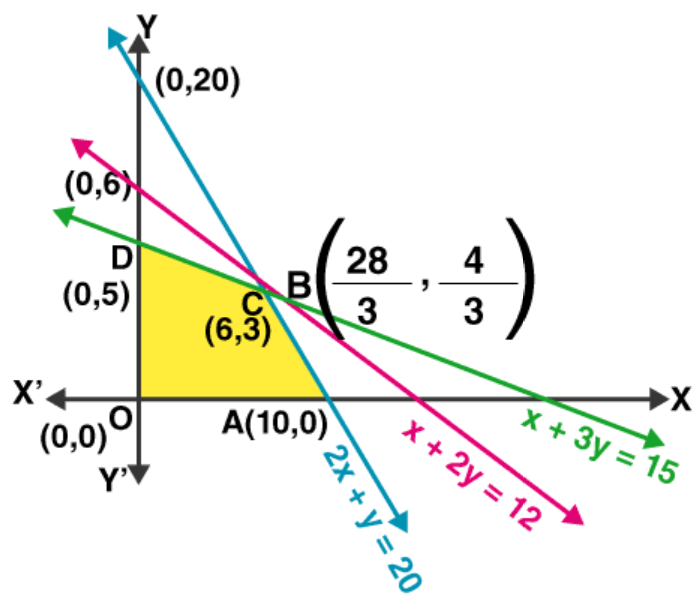As per the solution of exercise 11, we have

Maximize Z = 50x + 60y subject to the constraints

20x + 10y ≤ 200 2x + y ≤ 20 … (i)

10x + 20y ≤ 120 x + 2y ≤ 12 … (ii)

10x + 30y ≤ 150 x + 3y ≤ 15 … (iv)

x ≥ 0, y ≥ 0 … (iv)

Now, let’s construct a constrain table for the above

Table for (i)

 x 10 0 y 0 20

Table for (ii)

 x 12 0 y 0 6

Table for (iii)

 x 15 0 y 0 5

Next, solving equations (i) and (ii) we get,

x = 28/3, y = 4/3

So, the corner point is B(28/3, 4/3).

Solving equations (ii) and (iii) we get,

x = 6, y = 3 and the corner point is C(6, 3)

Lastly, solving equations (i) and (iii) we get,

x = 9, y = 2 (not included in the feasible region)

Here, OABCD is the feasible region.

Hence, the corner points are O(0, 0), A(10, 0), B(28/3, 4/3), C(6, 3) and D(0, 5).

Let us evaluate the value of Z

 Corner points Corresponding value of Z = 50x + 60y O(0, 0) Z = 50(0) + 60(0) = 0 A(10, 0) Z = 50(10) + 60(0) = 500 B(28/3, 4/3) Z = 50(28/3) + 60(4/3) = 1400/3 + 240/3 = 1640/3 = 546.6 C(6, 3) Z = 50(6) + 60(3) = 480 D(0, 5) Z = 50(0) + 60(5) = 300

So here, the maximum profit is Rs 546.6 which is not possible for number of items in fraction.

Therefore, the maximum profit for the manufacture is Rs 480 at (6, 3) i.e. Type A = 6 and Type B = 3.

17. Refer to Exercise 12. What will be the minimum cost?

Solution:

As per the solution of exercise 12, we have

The objective function for minimum cost is Z = 400x + 200y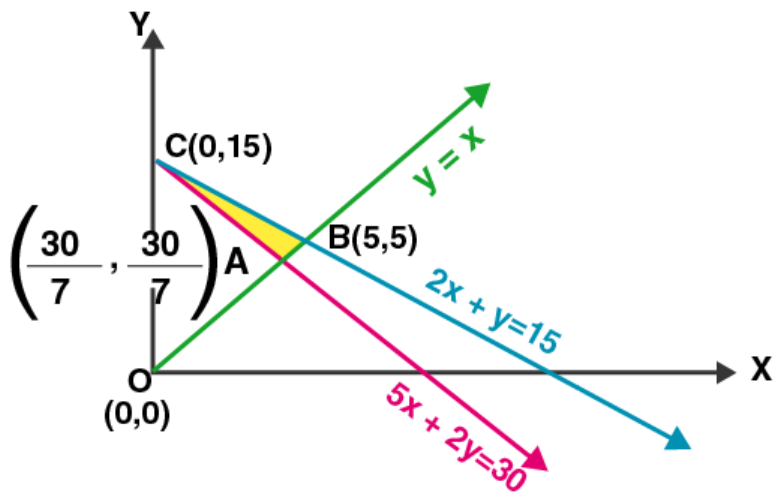Subject to the constrains;

5x + 2y ≥ 30 ….. (i)

2x + y ≤ 15 ….. (ii)

x ≤ y …. (iii)

and x ≥ 0, y ≥ 0 (non-negative constraints)

Now, let’s construct a constrain table for the above

Table for (i)

 x 0 6 y 15 0

Table for (ii)

 x 0 7.5 y 15 0

Table for (iii)

 x 1 0 y 1 0

Next, solving equations (i) and (iii), we get

x = 30/7 and y = 30/7, so the corner point is A(30/7, 30/7)

On solving equations (ii) and (iii), we get

x = 5 and y = 5, so the corner point is B(5, 5)

Here, ABC is the shaded feasible region whose corner points are A(30/7, 30/7), B(5, 5) and C(0, 15)

On evaluating the value of Z, we have

 Corner point Value of Z = 400x + 200y A(30/7, 30/7) Z = 400(30/7) + 200(30/7) = 18000/7 = 2571.4 B(5, 5) Z = 400(5) + 200(5) = 3000 C(0, 15) Z = 400(0) + 200(15) = 3000

From the table it’s seen that the minimum value is 2571.4

Therefore, the required minimum cost is Rs 2571.4 at (30/7, 30/7)

18. Refer to Exercise 13. Solve the linear programming problem and determine the maximum profit to the manufacturer.

Solution: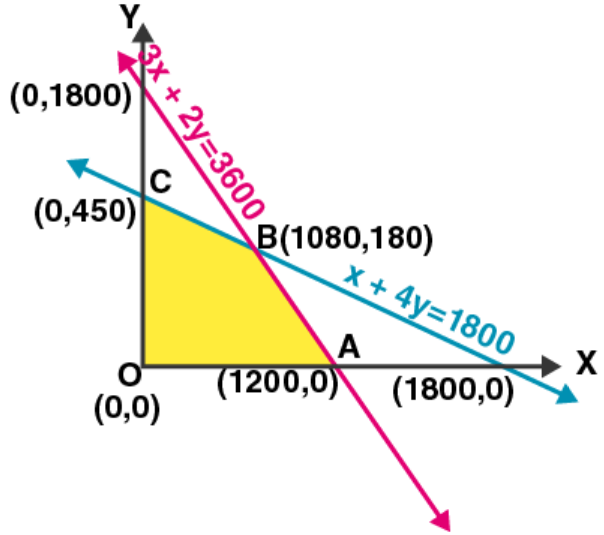As per the solution of exercise 13, we have

The objective function for maximum profit Z = 100x + 170y

Subject to constraints,

x + 4y ≤ 1800 …. (i)

3x + 2y ≤ 3600 … (ii)

x ≥ 0, y ≥ 0

Now, let’s construct a constrain table for the above

Table for (i)

 x 0 1800 y 450 0

Table for (ii)

 x 0 1200 y 1800 0

Next, solving equations (i) and (ii), we get

x = 1080 and y = 180

It’s seen that OABC is the feasible region whose corner points are O(0, 0), A(1200, 0), B(1080, 180) and C(0, 450).

On evaluating the value of Z, we have

 Corner points Value of Z = 100x + 170y O(0, 0) Z = 100(0) + 170(0) = 0 A(1200, 0) Z = 100(1200) + 170(0) = 120000 B(1080, 180) Z = 100(1080) + 170(180) = 138600 C(0, 450) Z = 100(0) + 170(450) = 76500

Form the table it’s seen that the maximum value of Z is 138600.

Therefore, the maximum profit of the function Z is 138600 at (1080, 180).

19. Refer to Exercise 14. How many sweaters of each type should the company make in a day to get a maximum profit? What is the maximum profit?

Solution:

As per the solution of exercise 14, we have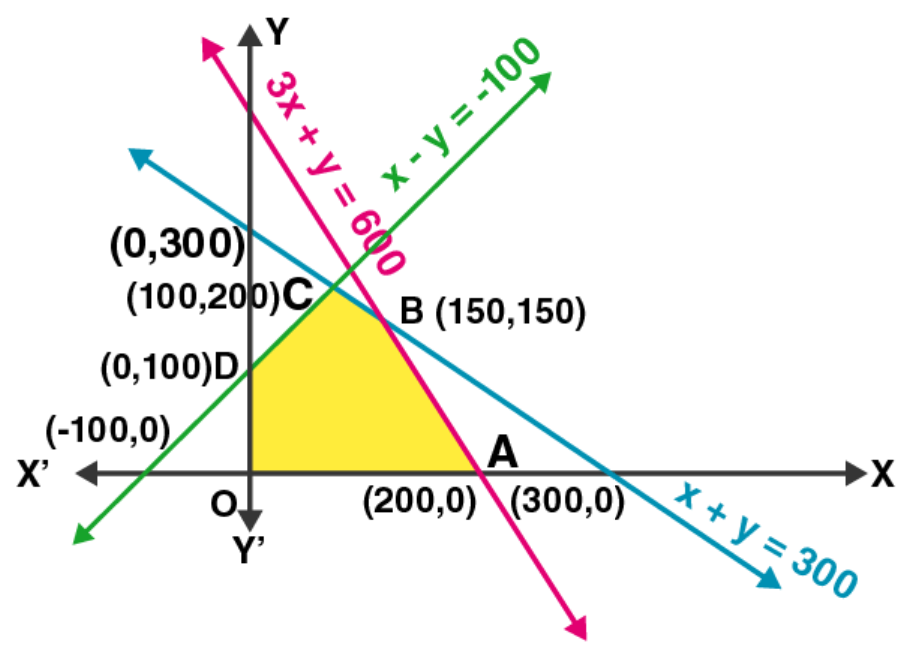Maximize Z = 200x + 120y subject to constrains

3x + y ≤ 600 …. (i)

x + y ≤ 300 …. (ii)

x – y ≤ -100 …. (iii)

x ≥ 0, y ≥ 0

Now, let’s construct a constrain table for the above

Table for (i)

 x 0 200 y 600 0

Table for (ii)

 x 0 300 y 300 0

Table for (iii)

 x -100 0 y 0 100

Next, solving equation (i) and (iii) we get

x = 100 and y = 200

On solving equation (i) and (ii), we get

x = 150 and y = 150

It’s seen that the shaded region is the feasible region whose corner points are O(0, 0), A(200, 0), B(150, 150), D(0, 100).

Evaluating the value of Z, we have

 Corner points Value of Z = 2000x + 120y O(0, 0) Z = 200(0) + 120(0) = 0 A(200, 0) Z = 200(200) + 120(0) = 40000 B(150, 150) Z = 200(150) + 120(150) = 48000 C(100, 200) Z = 200(100) + 120(200) = 44000 D(0, 100) Z = 200(0) + 120(100) = 12000

From the above table it’s seen that the maximum value is 48000.

Therefore, the maximum value of Z is 48000 at (150, 150) which means 150 sweaters of each type.

20. Refer to Exercise 15. Determine the maximum distance that the man can travel.

Solution:

As per the solution of exercise 15, we have

Maximize Z = x + y, subject to the constraints

2x + 3y ≤ 120 … (i)

8x + 5y ≤ 400 … (ii)

x ≥ 0, y ≥ 0

Now, let’s construct a constrain table for the above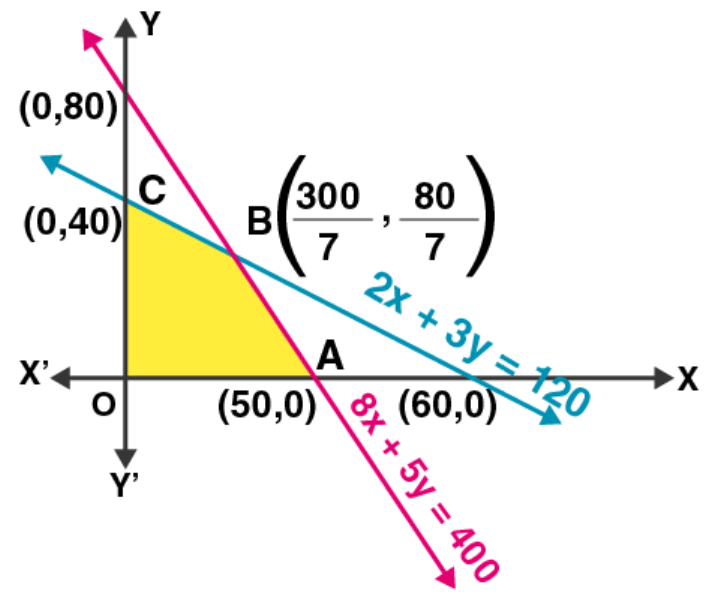Table for (i)

 x 0 60 y 40 0

Table for (ii)

 x 0 50 y 80 0

Next, solving equation (i) and (iii) we get

x = 300/7 and y = 80/7

It’s seen that the feasible region whose corner points are O(0, 0), A(50, 0), B(300/7, 80/7) and C(0, 40).

Let’s evaluate the value of Z

 Corner points Value of Z = x + y O(0, 0) Z = 0 + 0 = 0 A(50, 0) Z = 50 + 0 = 50 B(300/7, 80/7) Z = 300/7 + 80/7 = 380/7 = 54.3 C(0, 40) Z = 0 – 40 = 40

From above table the maximum value of Z is 54.3

Therefore, the maximum distance that the man can travel is 54.3 km at (300/7, 80/7).

21. Maximize Z = x + y subject to
x + 4y £ 8, 2x + 3y £ 12, 3x + y £ 9, x ³ 0, y ³ 0.

Solution: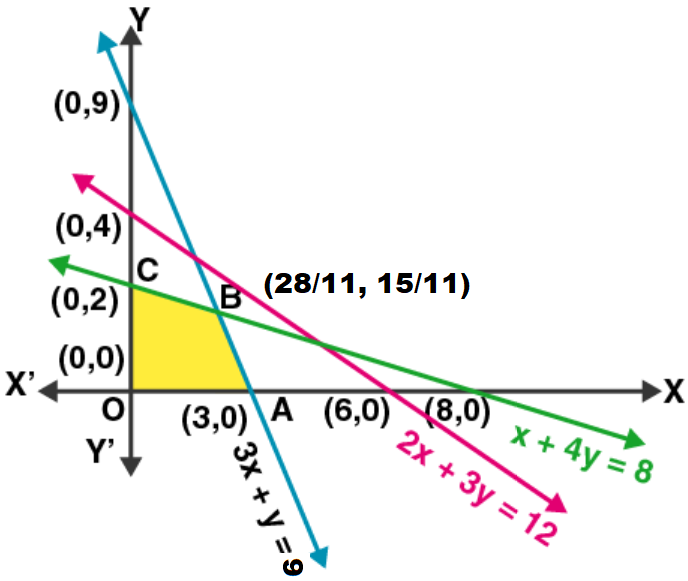Given: Z = x + y subject to constraints, x + 4y £ 8, 2x + 3y £ 12, 3x + y £ 9, x ³ 0, y ³ 0

Constructing a constrain table for the above, we have

Table for x + 4y = 8

 x 0 8 y 2 0

Table for 2x + 3y = 12

 x 0 6 y 4 0

Table for 3x + y = 9

 x 3 0 y 0 9

On solving equations x + 4y £ 8 and 3x + y £ 9, we get

x = 28/11 and y = 15/11

Here, it’s seen that OABC is the feasible region whose corner points are O(0, 0), A(3, 0), B(28/11, 15/11) and C(0, 2).

Now, let’s evaluate the value of Z

 Corner points Value of Z = x + y O(0, 0) Z = 0 + 0 = 0 A(3, 0) Z = 3 + 0 = 3 B(28/11, 15/11) Z = 28/11 + 15/11 = 43/11 = 3.9 C(0, 2) Z = 0 + 2 = 2

From the above table it’s noticed that the maximum value of Z is 3.9

Therefore, the maximum value of Z is 3.9 at (28/11, 15/11).

22. A manufacturer produces two Models of bikes – Model X and Model Y. Model X takes 6 man-hours to make per unit, while Model Y takes 10 man-hours per unit. There is a total of 450 man-hour available per week. Handling and Marketing costs are Rs 2000 and Rs 1000 per unit for Models X and Y respectively. The total funds available for these purposes are Rs 80,000 per week. Profits per unit for Models X and Y are Rs 1000 and Rs 500, respectively. How many bikes of each model should the manufacturer produce so as to yield a maximum profit? Find the maximum profit.

Solution:

Let’s take x an y to be the number of models of bike produced by the manufacturer.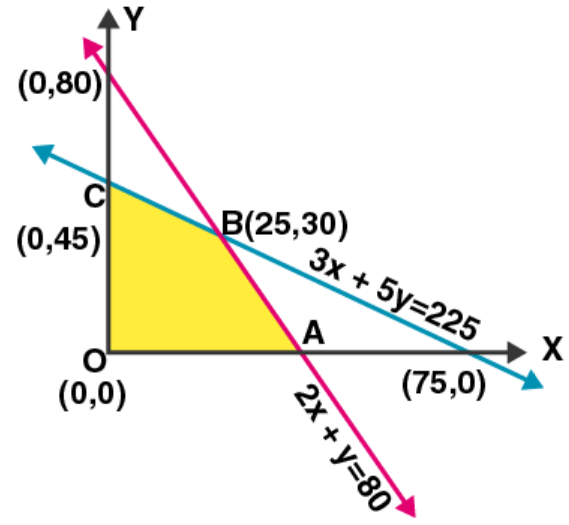From the question we have,

Model x takes 6 man-hours to make per unit

Model y takes 10 man-hours to make per unit

Total man-hours available = 450

So, 6x + 10y ≤ 450 ⇒ 3x + 5y ≤ 225 ….. (i)

The handling and marketing cost of model x and y are Rs 2000 and Rs 1000 respectively.

And, the total funds available is Rs 80,000 per week

So, 2000x + 1000y ≤ 80000 ⇒ 2x + y ≤ 80 … (ii)

And, x ≥ 0, y ≥ 0

Now, the total profit (Z) per unit of models x and y are Rs 1000 and Rs 500 repectively

⇒ Z = 1000x + 500y

Hence, the required LPP is

Maximize Z = 1000x + 500y subject to the constraints

3x + 5y ≤ 225, 2x + y ≤ 80 and x ≥ 0, y ≥ 0

Now, let’s construct a constrain table for the above:

Table for (i)

 x 75 0 y 0 45

Table for (ii)

 x 0 40 y 80 0

Next, on solving equation (i) and (ii) we get

x = 25 and y = 30

After plotting all the constraint equations, we observe that the feasible region is OABC, whose corner points are O(0, 0), A(40, 0), B(25, 30) and C(0, 45).

On evaluating the value of Z, we get

 Corner points Value of Z = 1000x + 500y O(0, 0) Z = 1000(0) + 500(0) = 0 A(40, 0) Z = 1000(40) + 500(0) = 40,000 B(25, 30) Z = 1000(25) + 500(30) = 40,000 C(0, 45) Z = 1000(0) + 500(45) = 22,500

Therefore, from the above table it’s seen that the maximum profit is Rs 40,000.

The maximum profit can be achieved by producing 25 bikes of model x and 30 bikes of model Y or by producing 40 bikes of model x.